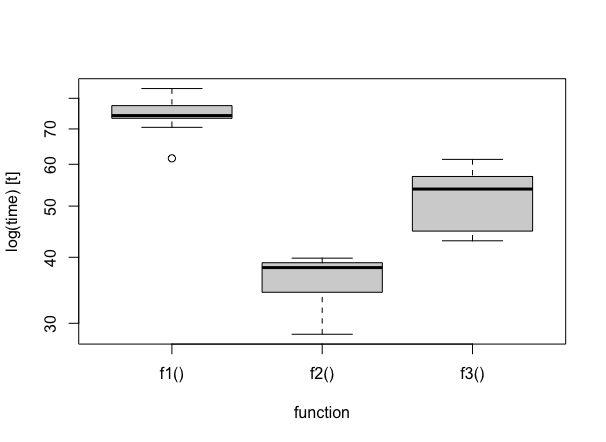# Install package ‘crossvalidation’

options(repos = c(
techtonique = 'https://techtonique.r-universe.dev',
CRAN = 'https://cloud.r-project.org'))

install.packages("crossvalidation")


# Import packages

library(crossvalidation)
library(randomForest)
library(microbenchmark)


# Input data

set.seed(123)
n <- 1000 ; p <- 10
X <- matrix(rnorm(n * p), n, p)
y <- rnorm(n)


# Random forest hyperparameters for a grid search

tuning_grid <- base::expand.grid(mtry = c(2, 3, 4),
ntree = c(100, 200, 300))
n_params <- nrow(tuning_grid)
print(tuning_grid)


# Sequential and parallel execution of cross-validation on a tuning grid

n_cores <- 4


## Sequential

f1 <- function() base::lapply(1:n_params,
function(i)
crossvalidation::crossval_ml(
x = X,
y = y,
k = 5,
repeats = 3,
fit_func = randomForest::randomForest,
predict_func = predict,
packages = "randomForest",
fit_params = list(mtry = tuning_grid[i, "mtry"],
ntree = tuning_grid[i, "ntree"])
))


## Parallel 1

f2 <- function() parallel::mclapply(1:n_params,
function(i)
crossvalidation::crossval_ml(
x = X,
y = y,
k = 5,
repeats = 3,
fit_func = randomForest::randomForest,
predict_func = predict,
packages = "randomForest",
fit_params = list(mtry = tuning_grid[i, "mtry"],
ntree = tuning_grid[i, "ntree"])
), mc.cores=n_cores)


## Parallel 2

f3 <- function() base::lapply(1:n_params,
function(i)
crossvalidation::crossval_ml(
x = X,
y = y,
k = 5,
repeats = 3,
fit_func = randomForest::randomForest,
predict_func = predict,
packages = "randomForest",
fit_params = list(mtry = tuning_grid[i, "mtry"],
ntree = tuning_grid[i, "ntree"]),
cl=n_cores
))


## Check that the three functions return the same result

all.equal(f1(), f2())
all.equal(f2(), f3())


## Timings for f1, f2, f3

(timings <- microbenchmark::microbenchmark(f1(), f2(), f3(),
times = 10L))


## Plot results:

boxplot(timings, xlab = "function")print(sessionInfo())

R version 4.0.4 (2021-02-15)
Platform: x86_64-apple-darwin17.0 (64-bit)
Running under: macOS Big Sur 10.16

Matrix products: default
LAPACK: /Library/Frameworks/R.framework/Versions/4.0/Resources/lib/libRlapack.dylib

locale:
 fr_FR.UTF-8/fr_FR.UTF-8/fr_FR.UTF-8/C/fr_FR.UTF-8/fr_FR.UTF-8

attached base packages:
 stats     graphics  grDevices utils     datasets  methods   base

other attached packages:
 microbenchmark_1.4-7  randomForest_4.6-14   crossvalidation_0.3.0
 foreach_1.5.1         forecast_8.14         httr_1.4.2

loaded via a namespace (and not attached):
 Rcpp_1.0.6        urca_1.3-0        pillar_1.4.6      compiler_4.0.4
 iterators_1.0.12  tseries_0.10-47   tools_4.0.4       xts_0.12.1
 digest_0.6.25     jsonlite_1.7.2    nlme_3.1-152      lifecycle_0.2.0
 tibble_3.0.3      gtable_0.3.0      lattice_0.20-41   doSNOW_1.0.19
 pkgconfig_2.0.3   rlang_0.4.10      rstudioapi_0.11   curl_4.3
 parallel_4.0.4    dplyr_1.0.2       xml2_1.3.2        generics_0.0.2
 vctrs_0.3.4       lmtest_0.9-38     grid_4.0.4        nnet_7.3-15
 tidyselect_1.1.0  glue_1.4.2        R6_2.5.0          snow_0.4-3
 crossval_0.2.1    farver_2.0.3      ggplot2_3.3.3     purrr_0.3.4
 TTR_0.24.2        magrittr_1.5      codetools_0.2-18  scales_1.1.1
 ellipsis_0.3.1    quantmod_0.4.17   mime_0.9          timeDate_3043.102